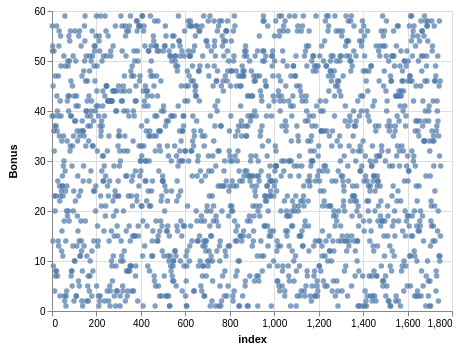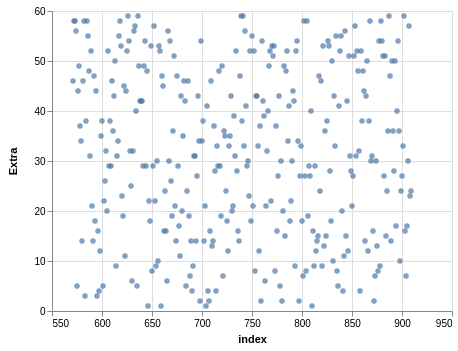# M.E. Irizarry-Gelpí

Physics impostor. Mathematics interloper. Husband. Father.

# Analyzing the New York Lotto

The State of New York has a website where you can find data produced by the state. Today I am going to use Pandas to explore a data set for the winning numbers of the New York Lotto. This is a lottery where six numbers are drawn (each between 1 and 59), followed by a bonus draw of a single number (also between 1 and 59).

The data file I have goes from 09/12/2001 to 06/20/2018 and it contains 1750 rows and 4 columns. First, you import Pandas and load the data into a `pd.DataFrame`:

``````import pandas as pd

``````

Using `.head()` gives you a short summary of the first five records. There is a column with the draw date (when the numbers where drawn), a column `Winning Numbers` with the six winning numbers (as a string), a column `Bonus #` with the bonus draw, and a column `Extra #` with an extra draw (which I do not understand).

You can rename some of the columns with `.rename`:

``````df = df.rename(columns={'Bonus #': 'Bonus', 'Extra #': 'Extra'})
``````

You can use `.describe()` to get a short description of the data. Here you learn that, out of the 1750 records, only 339 have a valid value in the `Extra` column. The statistics are encouraging: the mean value in both the `Bonus` and `Extra` column is between 30 and 32. The minimum and maximum values in both columns are 1 and 59, which means that at least these two numbers have been drawn in both cases. In the `Bonus` column, the first, second, and third quartiles are 15, 30, and 45, which suggests a uniform distribution. In the `Extra` column, the first, second, and third quartiles are 16.5, 31, and 47.

You can extract the `Bonus` column as follows:

``````bonus = pd.DataFrame()
bonus['Bonus'] = df['Bonus']
bonus = bonus.reset_index()
``````

The first line initializes an empty `pd.DataFrame`. The second line adds a column to `bonus` from `df`. The third line adds a column called `index`. The data in this column can be visualized via

``````bonus.plot.scatter(x='index', y='Bonus')
``````

Similar steps can be taken with the `Extra` column:

``````extra = pd.DataFrame()
extra['Extra'] = df['Extra']
extra = extra.dropna()
extra = extra.reset_index()
extra.plot.scatter(x='index', y='Extra')
``````

Here, the third line drops all of the rows with `NaN`.

You can use Altair for visualization:

``````import altair as alt
``````

For example, with the `Bonus` column you have:

``````alt.Chart(bonus).mark_circle().encode(
x='index',
y='Bonus',
)
``````

I encountered a problem when plotting the data in the `Extra` column, and solved that problem with the following code:

``````alt.Chart(extra).mark_circle().encode(
x=alt.X(
field='index',
type='quantitative',
scale=alt.Scale(zero=False),
),
y='Extra',
)
``````

You get the following two graphs:As you can tell, these scatter plots appear to be uniformly distributed, which is what you would expect for a fair lottery.

Another visualization tool is Seaborn. You can use Seaborn to great scatter plots with histograms:

``````import seaborn as sns

sns.jointplot(
x='index',
y='Bonus',
data=bonus,
)

sns.jointplot(
x='index',
y='Extra',
data=extra,
)
``````

Again, these histograms show that the distribution is almost uniform. In a future post I will look at the winning numbers.